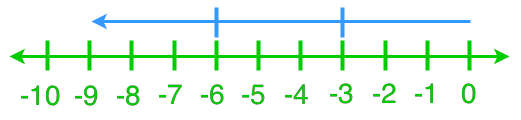Dividing integers

Dividing integers

In this section, we will keep working on dividing integers. We will learn how to read and represent division statements on a number line. We will then practice more integer divisions with actual numbers and word problems.

Lessons

In this lesson, we will learn:

• Understanding Division Using a Number Line
• Solving Division Statements Using a Number Line
• Division Statements With Integer Quotients
• Division Statements With Non-Integer Quotients
• Word Problems of Dividing Integers

Notes:
• Recurring/ repeating decimals: decimal numbers whose digits repeat forever.
• For the recurring decimals, we put either a bar or dots above the recurring parts.
• Introduction
Introduction to division statements with non-integer quotients

• 1.
Understanding Division Using a Number Line
What are the two division statements that each diagram represents?
a)b)• 2.
Solving Division Statements Using a Number Line
Use a number line to find each quotient.
a)
(+10)÷(+5)

b)
(+8)÷(-2)

c)
(-12)÷(+4)

d)
(-9)÷(-3)

• 3.
Division Statements With Integer Quotients
Solve.
a)
(+36)÷(-6)

b)
(-45)÷(+15)

c)
(-28)÷(-4)

d)
0÷(-16)

• 4.
Division Statements With Non-Integer Quotients
Solve.
a)
(-36)÷(+8)

b)
(-100)÷(-3)

c)
(+47)÷(+6)

d)
(+34)÷(-7)

• 5.
Word Problems of Dividing Integers
Katie participated in a track competition. If her average sprinting speed is 8 m/s, how long does it take for her to finish an 100 m sprint?

• 6.
Peter finished a 45 km long cycling trail. He first cycled for 3 hours and then, he took a break. Afterwards Peter cycled for another 2 hours to finish the trail. What was his cycling rate per hour? Were there any assumptions?